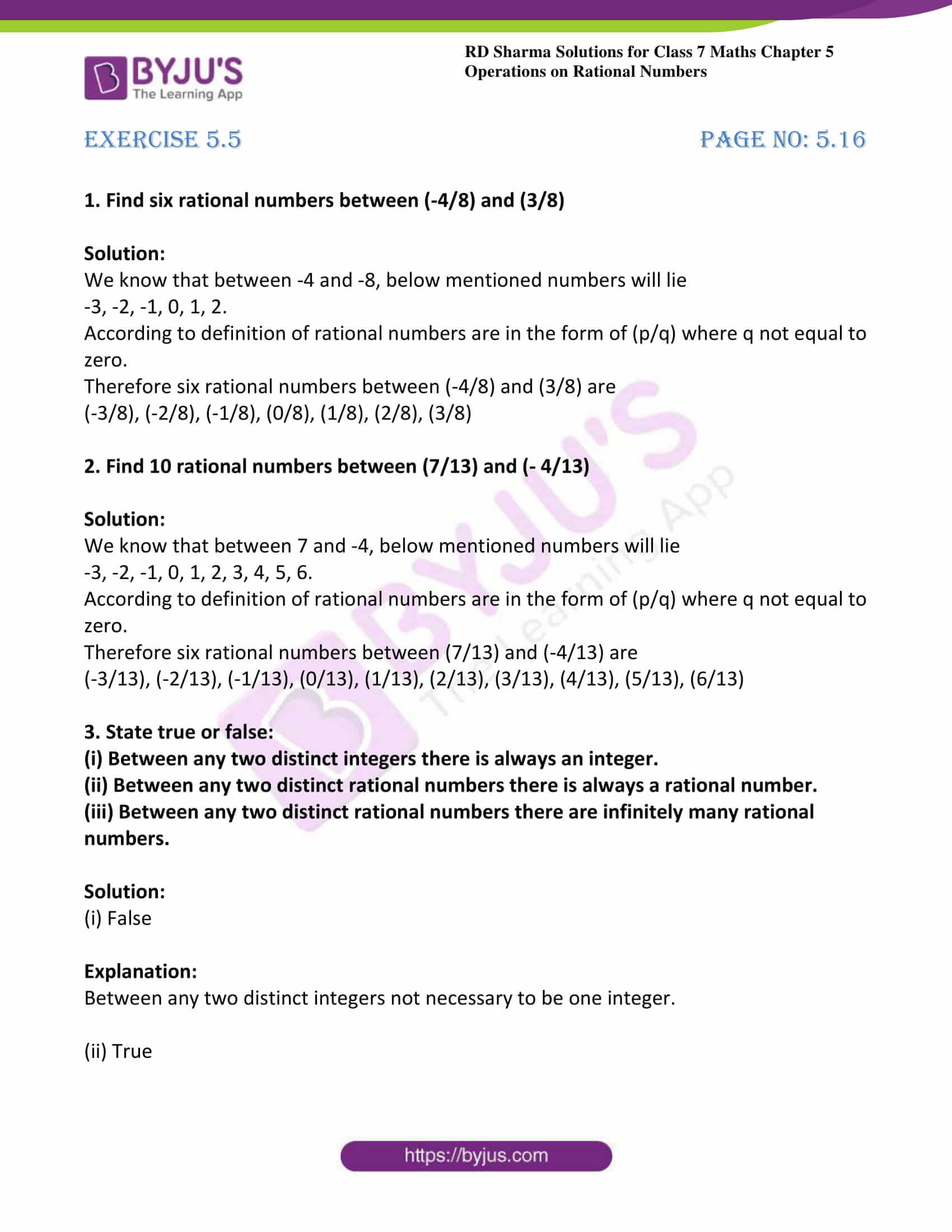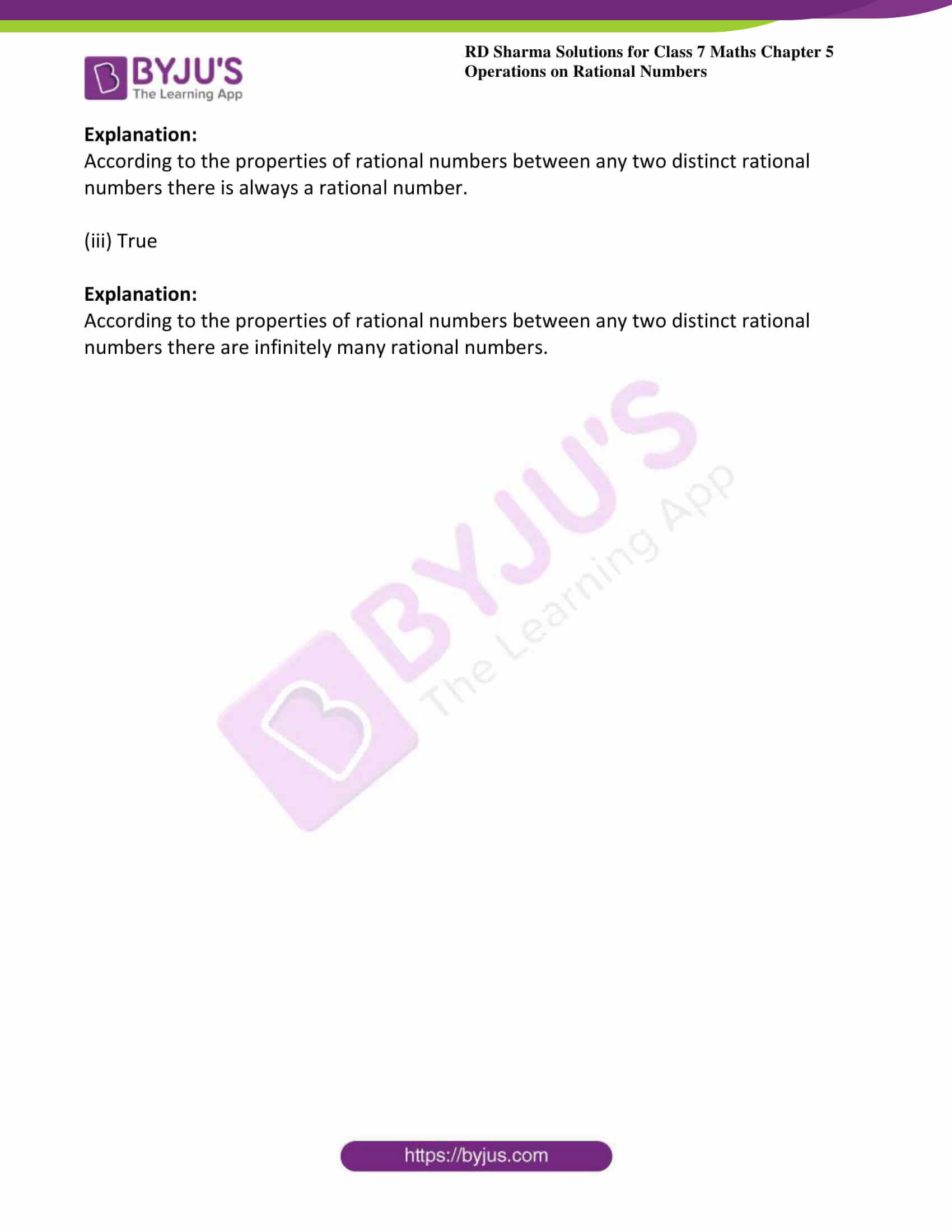# RD Sharma Solutions For Class 7 Maths Exercise 5.5 Chapter 5 Operations On Rational Numbers

Students can refer and download the RD Sharma Solutions for Class 7 Maths Exercise 5.5 of Chapter 5 from the links provided below. The syllabus given by the CBSE for all the classes is completely based on the RD Sharma textbook. Hence, preparation of exams using the RD Sharma Solutions for Class 7 will definitely provide students with an advantage of scoring well. This exercise includes the insertion of rational numbers between two given rational numbers. This property of rational numbers is known as dense property.

## Download the PDF of RD Sharma Solutions For Class 7 Maths Chapter 5 – Operations On Rational Numbers Exercise 5.5### Access answers to Maths RD Sharma Solutions For Class 7 Chapter 5 – Operations On Rational Numbers Exercise 5.5

1. Find six rational numbers between (-4/8) and (3/8)

Solution:

We know that between -4 and -8, below mentioned numbers will lie

-3, -2, -1, 0, 1, 2.

According to definition of rational numbers are in the form of (p/q) where q not equal to zero.

Therefore six rational numbers between (-4/8) and (3/8) are

(-3/8), (-2/8), (-1/8), (0/8), (1/8), (2/8), (3/8)

2. Find 10 rational numbers between (7/13) and (- 4/13)

Solution:

We know that between 7 and -4, below mentioned numbers will lie

-3, -2, -1, 0, 1, 2, 3, 4, 5, 6.

According to definition of rational numbers are in the form of (p/q) where q not equal to zero.

Therefore six rational numbers between (7/13) and (-4/13) are

(-3/13), (-2/13), (-1/13), (0/13), (1/13), (2/13), (3/13), (4/13), (5/13), (6/13)

3. State true or false:

(i) Between any two distinct integers there is always an integer.

(ii) Between any two distinct rational numbers there is always a rational number.

(iii) Between any two distinct rational numbers there are infinitely many rational numbers.

Solution:

(i) False

Explanation:

Between any two distinct integers not necessary to be one integer.

(ii) True

Explanation:

According to the properties of rational numbers between any two distinct rational numbers there is always a rational number.

(iii) True

Explanation:

According to the properties of rational numbers between any two distinct rational numbers there are infinitely many rational numbers.